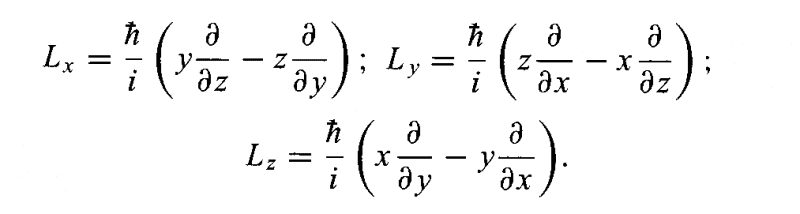# Commutatos and angular momentum

• Monalisa

## Homework Statement

Let e and f be unit vectors. Le = eL is the definition of the component of angular momentum in direction e. Calculate the commutator [Le,Lf ] in terms of e, f and L

[A,B]=(AB-BA)

## The Attempt at a Solution

we know that L=r x p, in classical mechanics, and in quantum physics we have the operators for angular momentum in cartesian coordinates for example, but in my problem I have just two direction, e and f, and I am obtaining as answering 0. How can I do this exercise ? thanks